Level 8

32.    (a)     An early type of echo sounder for a boat produces a sound of frequency 2000 Hz.

(i)      The wavelength of this sound in water is 0.75 m.  What is the speed of the sound in water? Give the unit.

.

1 mark

(ii)     This sound is heard by the operator as a brief ‘ping’.  The speed of sound in air is 330 m/s.  What is the wavelength of the sound in air? Give the unit.

.

1 mark

(iii)     Modern echo sounders use higher frequencies than the early type.  Complete the sentence below to describe how the wavelength produced by a modern echo sounder compares with that produced by the early type.

The wavelength produced by a modern echo sounder is

1 mark

(b)     The diagram shows a wave in a ‘slinky’ spring.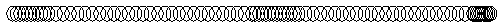The frequency of the waves is 5 pulses per second and the wavelength is 80 cm.

What is the wave speed? Give the unit.

.

1 mark

33.    A writer of science fiction likes to make her stories as realistic as possible.  In her latest book there is a group of space travellers who discover a star in a distant part of the galaxy.  The travellers are excited to find a planet very similar to Earth orbiting this star.  They call the planet Delta.

The table compares data about Delta and the Earth.  Most of the figures are relative.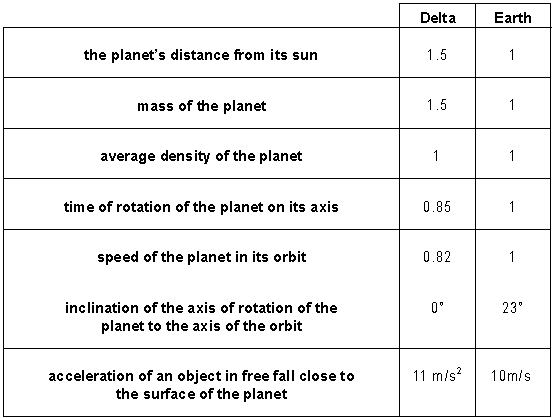(a)     In her story, how should the writer compare conditions on Delta with those on Earth? Tick the correct box in each case.

greater       less        same

length of year

length of day

radius of the planet

3 marks

(b)     One of the space travellers lands on planet Delta.  With her space suit, she has a mass of 100 kg.

What is her weight on planet Delta? Give the unit.

.

1 mark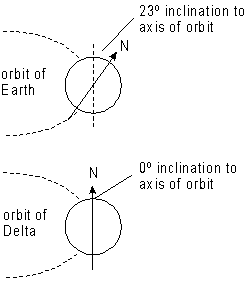(c)     The inclination of the axis of rotation to the axis of orbit is different for Delta and Earth.  In the story, the travellers spend a year on Delta.  Describe two differences between Delta and Earth that the travellers would find, apart from their weight.

1.

1 mark

2.

1 mark

34.    (a)     Satellites orbiting the Earth appear as points of light in the night sky. They look similar to stars. An astronomer sees a point of light in the night sky.

(i)      How can she tell that the point of light is a satellite in a low orbit and not a star?

1 mark

(ii)     She looks through a telescope and sees another point of light which is a satellite in a geostationary orbit. How can she tell that this satellite is in a geostationary orbit?

1 mark

(b)     The Hubble Space Telescope is a telescope used to observe the planets and stars. It is in a satellite orbiting close to the Earth.

(i)      Give one reason why a telescope in orbit gives clear pictures.

1 mark

(ii)     Give one disadvantage of an astronomical telescope in orbit round the Earth.

1 mark

(c)     In 1994, astronauts visited The Hubble Space Telescope to repair it. Astronauts have to train themselves to move objects in a controlled way in space.

Name a pair of antagonistic muscles which has to be controlled.

1 mark

35.    The diagram shows a magnetic lock for a door. It consists of both a strong permanent  magnet and an electromagnet. It is fitted into the door frame. An iron plate is attached to the door.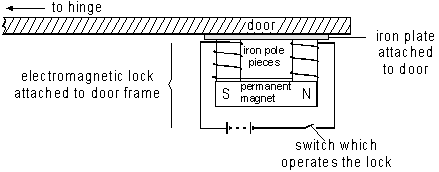(a)     In the diagram the switch is open. Explain why the door cannot be opened.

1 mark

(b)     When the switch is closed the door may be opened. Explain why the door may now be opened.

1 mark

(c)     A burglar tries to get in by cutting the wires to the battery. Explain why the door still cannot be opened.

1 mark

(d)     When the wires are repaired, the battery is accidentally connected the other way round.

Explain why the door cannot now be opened.

1 mark

(e)     Another magnetic lock is shown below. It does not have a permanent magnet in it.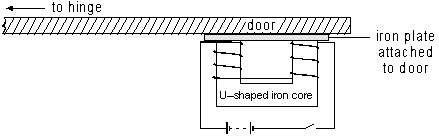Explain how this kind of lock works and why it is not as secure as the one shown at the beginning of the question.

3 marks

36.    The graph shows the results of a test in which a car accelerates to its maximum speed.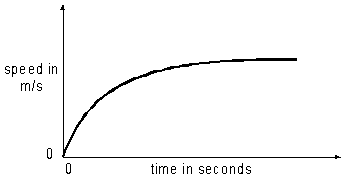(a)     (i)      Describe how the acceleration of the car changes after the car has started to move.

1 mark

(ii)     How does the resultant force on the car change?

1 mark

The car has a mass of 1000 kg and the maximum forward force on the car, produced by the engine, is 4000 N.

It is claimed that the car will accelerate from 0 to 24 m/s in 6 seconds.

(b)     Use calculations, with the correct units, to show that the claim is false.

3 marks

37.    (a)     An electrical current is a flow of charge.  The diagram shows a circuit containing six identical bulbs.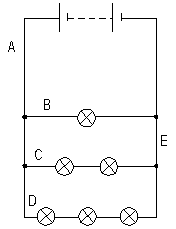In which part of the circuit, A, B, C, D or E, is there:

(i)      the greatest flow of charge?

(ii)     the least flow of charge?

2 marks

(b)     Sulphur is an electrical insulator, but it can be made to conduct electricity if a very bright light shines on it.

(i)      A slab of sulphur is rubbed with a piece of woollen cloth.
Explain how this gives the sulphur a negative electrical charge.

1 mark

(ii)     A very bright light shines onto a small circular area in the centre of the charged slab of sulphur.  The pattern of charge on the sulphur alters.  The simplified diagram represents the new pattern of charge.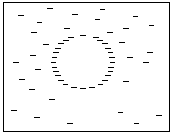Explain why the pattern alters in this way.

1 mark

38.    Digital information can be stored on magnetic recording tape in several different ways.  One way is shown in the diagram below.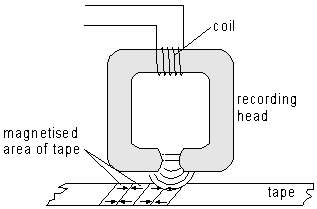The recording head produces a magnetic field.  The magnetic particles in the section of tape under the recording head line up with the field.

(a)     As the tape moves past the recording head, different sections of the tape become magnetised.  The direction of the current is changed at regular intervals.

How would the pattern on the tape be different if the tape were moved past the recording head more quickly?

1 mark

(b)     The direction of the current is changed at regular intervals. The tape is moved past the recording head at a steady speed.

The gap between the poles of the recording head is made much bigger. Suggest two effects this might have on the pattern on the tape.

1

2

2 marks

39.    The diagram shows the Earth’s orbit. The Earth is shown in two positions six months apart. In one of the positions it is midsummer in Britain, and in the other positions it is midwinter.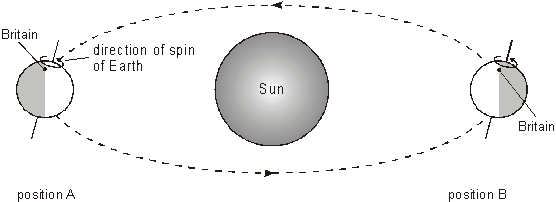(a)     Estimate what time of the day it is in Britain when the Earth is in position B, and explain your answer.

1 mark

(b)     The Earth has rotated exactly 183 times on its axis as it moved between the two positions shown.

(i)      Explain how you can tell from the diagram that the Earth has rotated a whole number of times.

1 mark

(ii)     Explain how you can tell from the diagram that the time of day in Britain is different in the two positions.

1 mark

(iii)     While the Earth has rotated exactly 183 times, only 182.5 days have passed.
Calculate the exact time taken for each rotation of the Earth on its axis.

1 mark

(c)     The Moon orbits the Earth approximately once each month. However, only one face of the Moon is ever visible from the Earth.

(i)      Explain why we always see the same face of the Moon.

1 mark

(ii)     Suggest how long in Earth days the period from sunrise to sunrise will be at a point on the Moon’s surface.

1 mark

40.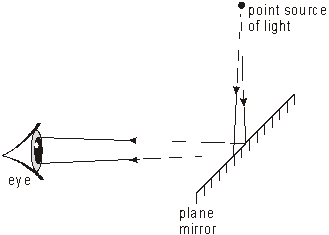The diagram shows rays of light coming from a point source, reflecting in a plane mirror, and entering a person's eye. The person sees an image of the light source at a point behind the mirror.

(a)     On the diagram, draw construction lines to find the position where the image appears. Label the image I.

1 mark

(b)     (i)      The person moves further away from the mirror.
How does this affect the position of the image relative to the mirror?

1 mark

(ii)     The point source of light is moved closer to the mirror, How does this affect the position of the image?

1 mark

The diagram shows two rays of light coming from a small fish in some water. The rays enter a person's eye.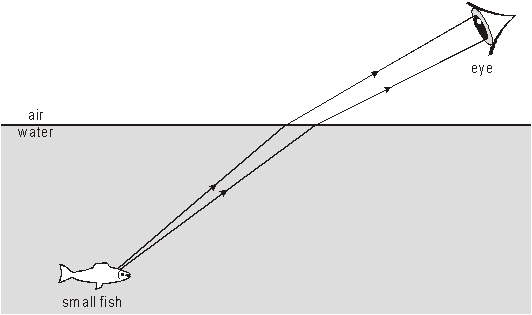(c)     The person sees an image of the fish under the water.
On the diagram, draw construction lines to find the position of the image.
Label the image
I.

1 mark

(d)     In some parts of the world, people catch fish using spears.
When they see the image of a fish in the water, where should they aim?

1 mark

41.    The simplified diagram shows a device which protects people from being electrocuted when using power tools such as hedge cutters. The wire in one part of the circuit is shown darker to make the circuit clearer.

When the armature is attracted towards the soft iron core, the contacts are pushed apart and break the circuit.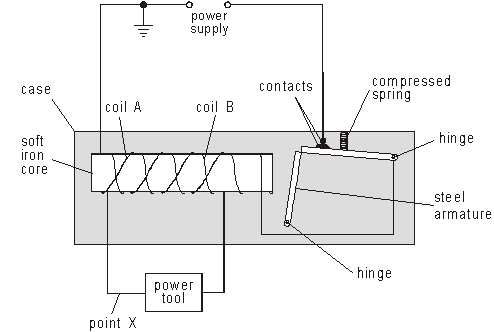(a)     The power tool, coil A and coil B are all in the same series circuit. Coils A and B are wound in opposite directions.

The current in the coils has no magnetic effect on the armature. Explain why.

1 mark

(b)     A gardener accidentally cuts the wire at point X. Current from the power supply starts to flow through the gardener to earth.

What effect will this have on the armature? Explain your answer.

3 marks